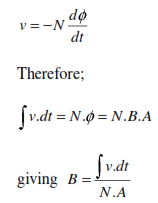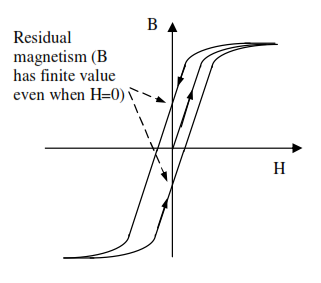## Magnetic permeability and the b/h curve, Electrical Engineering

Assignment Help:

Magnetic permeability and the B/H curve

To measure the relationship between field strength H and flux density B for a material, a sample is made into a closed ring and a magnetising coil wound onto it. Current flowing in this coil creates a m.m.f. equal to NI which in turn produces a magnetic field

H= NI/L

in the ring. So  H is proportional to the current in the energising coil and may be obtained from direct measurement of I. If a change in the current occurs, the flux density changes and this will induce a transient 'back e.m.f' in a second coil that is also wound onto the ring.The integral of the back emf is proportional to B. Hence the relationship between B and H may be determined from measurement of I (to give H) and the induced back e.m.f in the coils (to give B).

The result of such measurements on various materials produces two types of curves - straight lines of low

B/H =µr

values and curves for  which the value of B/H =µr   varies. This first type are sub divided into paramagnetic
varies. This first type are sub divided into paramagnetic materials if B/H =µr >1 and diamagnetic if B/H =µr <1. The second type are ferromagnetic and have a more complicated dependence of B on H.  At low H these have B/H =µ>>1 (typically 100 - 100000)It is also found that ferromagnetic materials lose these high µr properties above a well-defined temperature (different for different materials) known as the Curie temperature. This typically  r ranges from 200 - 750 °C and limits the maximum temperature that many electromagnetic devices can operate.

The temperature dependence of the ferromagnetic properties give a clue to the physical reason for the different

permeabilities of the materials. Diamagnetic and paramagnetic properties are attributable to the orbiting electrons within the atoms of the material. Ferromagnetic properties arise due to the orientation of atoms on a macroscopic scale so that the magnetic fields created by the orbiting electrons within each atom reinforce each other. These 'domains' of high magnetic strength may contain ~10-12   atoms each. The domains are initially randomly directed but under the influence of an external magnetic field, re-orientate to point in the same direction. Initially this is along the direction of the nearest crystal axis to the direction of the magnetic field.

Steadily increasing the external field brings them directly into line with the external field direction.If the external field is now removed, the domains relax back to point in the nearest crystal direction, leaving a residual magnetism. Materials that have a strong residual magnetism are used for permanent magnets. Cycling the current over one complete cycle produces a hysteresis B/H curve#### Why do we use xra a instruction, Why do we use XRA A instruction The XR...

Why do we use XRA A instruction The XRA A instruction is used to clear the contents of the Accumulator and keep the value 00H.

#### Find the sending end voltage and sending end current, A 3-phase transmissio...

A 3-phase transmission line is 200km long. The line has a per phase series impedance of 0.25+j0.45 Ω/km and shunt admittance of j7.2 μS/km. The line delivers 250MVA, at 0.6 lagging

#### Illustrate balanced delta-connected load, Next, let us consider the case of...

Next, let us consider the case of a balanced delta-connected load with impedance of 5 45° supplied by a three-phase, three-wire 100-V system, as shown in Figure (a). We shall dete

#### Split-phase or resistance-split-phasemotor, Q. Split-phase or resistance-sp...

Q. Split-phase or resistance-split-phasemotor? Split-phase or resistance-split-phasemotors: Split-phasemotors have two statorwindings (amainwinding and an auxiliarywinding)with

#### Electric Optic Device, Transmission Through a LiNb0 3 Plate Examine the tr...

Transmission Through a LiNb0 3 Plate Examine the transmission of an unpolarized He-Ne laser beam (?o= 633 nm) normally incident on a LiNb0 3 plate (ne = 2.29, no = 2.20) of thickn

#### Jfet, JFET The JFET (junction gate field-effect transistor) is a ...

JFET The JFET (junction gate field-effect transistor) is a long channel of semiconductor material, doped to consist of abundance of positive charge carriers (p-type), or

#### #title.energy harvesting from door movement., How to convert mechanical ene...

How to convert mechanical energy into electrical energy

#### Explain contact resistance, Explain Contact resistance. Contact resista...

Explain Contact resistance. Contact resistance: It is measured as the voltage drop from tail to tail of the mated contacts along with specified current flowing through the cont

#### Linear system to a unit-step excitation, Q. The response v(t) of a linear s...

Q. The response v(t) of a linear system to a unit-step excitation i(t) is given by v(t) = (5 - 3e -t + 2e -2t ) u(t). Determine the transfer function H(s) = V (s)/I (s).

#### Compareson, compear copper and aluminum conductore in termes of price ,weig...

compear copper and aluminum conductore in termes of price ,weight,resistance for equal length .